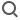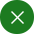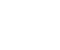SearchEnglish
Electrical transport measurements

Electrical transport measurements are a fundamental materials characterization technique that can provide insight into the scattering mechanisms and band structure of solid-state materials. As described in quantum mechanics, macroscopic carrier transport is one of the most fundamental concepts of electronic material properties, with significant gate-tunable effects in low-dimensional systems and at low temperatures.

Common 2-terminal and 4-terminal measurement configurations . Electrical, optical, or thermal excitation is applied to the sample , and the excitation is converted into a voltage (V) or current (I) signal. These measurements are usually made at low frequencies with significant background noise.

measurement method depends on the impedance of the sample and the shape of the sample. AC techniques using lock-in amplifier amplifiers provide higher sensitivity, signal-to-noise ratio and dynamic range, and faster measurements than DC measurements, which are also prone to larger systematic errors.

### 2 -terminal method

2 -terminal method measurement is usually carried out at constant voltage to measure the conductivity characteristics of the studied sample . An example of such a measurement is through the conductance properties of carbon nanotubes , where a constant voltage is applied to the sample and conductance is measured as I(measured)/V(applied). The 2-terminal measurement is easy to operate and can be used in the following scenarios:

• The contact resistance is negligible relative to the sample resistance.

•   External excitation of the sample by optical or other means.

### 4 -terminal method

4 -terminal method measurement is performed at a constant current and the sample to be tested is calculated as the voltage across the sample divided by the current flowing through the sample .

The constant current is provided by a constant current source or a current limiting resistor; in the case of a current limiting resistor, the resistance value of the current limiting resistor needs to be much larger than the sampling resistor (impedance) .

4 -terminal measurements can be made in Hall bar or Vanderburgh geometry. Compared with the 2 -terminal measurement, the setup of the 4 -terminal method is more complicated. If it is one of the following conditions, the 4 -terminal method is preferred.

• The impedance of the sample is small.

•  contact resistance of the material is relatively high .

Apply for probation